﻿﻿沉井不排水下沉施工方案

（一）、概述

（二）、施工工艺流程

（三）、吊装前施工准备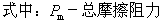β－附加阻力系数，β1.5

P－平台自重和工作荷载， P70+450520KN

μ－轴承滚动摩擦系数 ，μ0.015

d－滚道直径，d200mm

f－行走轮滚动摩擦系数，f0.5

D－行走轮直径，D400mm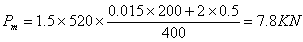6、钢制平台安装

（四）、起重机械选型

≥42t +0.7t=42.7t

Q1－抓铲机的重量；

Q2－索具的重量，取700kg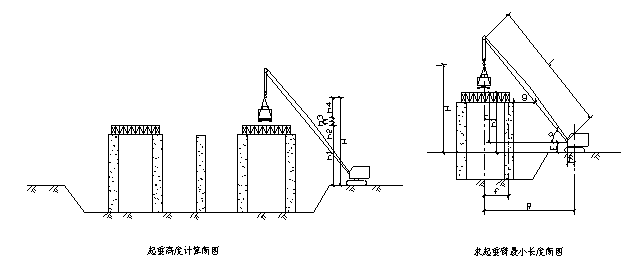2）起重高度

H≥h1+h2+h3+h4

h1——安装支座表面的高度（m），从停机面算起；

h2——安装空隙，不小于0.3m

h3——绑扎点至所吊抓铲机底面的距离（m）。

h2——索具高度（m），自绑扎点至吊钩面；

H≥h1+h2+h3+h4

≥9+0.5+2+4.5=16m

3）起重半径

L≥L1+L2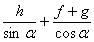h——起重机下铰至吊装支座的高度（m）；

h=h1E

f——起重钩需跨过结构的距离（m）；

g——起重臂轴线与已完成结构间的水平距离，至少取1m

α——起重臂的仰角；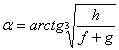f=3.3mg=1.2mE=1.5mh=h1-E=8.8-1.5=7.3m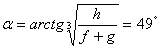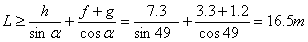4）起重半径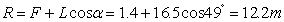（五）、活动平台强度、刚度校核

1、活动平台强度校核

（1）、活动平台受力分析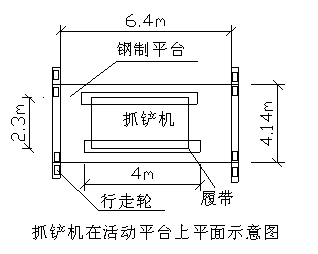2）、强度校核

钢材为Q235钢，许用应力 [σ]=160Mpa，焊条采用J422型，许用应力[σ]=160Mpa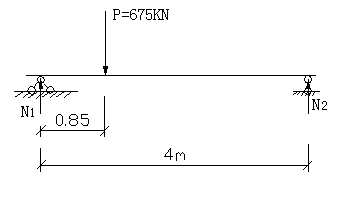N2=0.85/4×P=143K

N1=P-N2=532KN

N1=qmax×6.7×1/2

∴qmax=2×532/6.7=158.8KN/m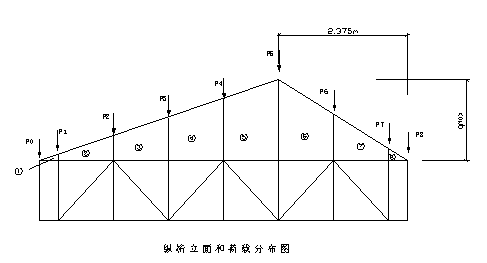s=1/2×12.8×0.35=2.24KN

s=1/2×12.8+49.568×1=31.184KN

s=1/2×49.568+86.28×1=67.924KN

s=1/2×86.28+123×1=104.64KN

s=1/2×123+158.8×1=140.9KN

s=1/2×90.09+158.8×1=124.045KN

s=1/2×23.35+90.09×1=56.72KN

s=1/2×23.35×0.35=4.086KN

ΣS=532.1KN=N1

P0=1/2 s=1.12KN

P1=1/2s+ s=16.712KN

P2=1/2s+ s=49.554KN

P3=1/2s+ s=86.282KN

P4=1/2s+ s=122.77KN

P5=1/2s+ s=132.472KN

P6=1/2s+ s=90.38KN

P7=1/2s+ s=30.403KN

P8=1/2s=2.043KN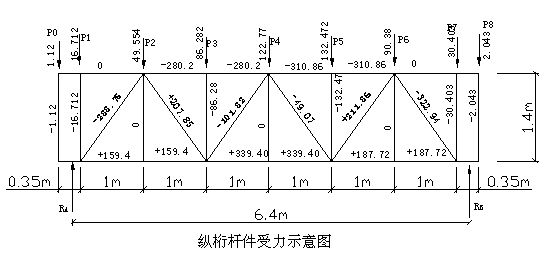a、求支座反力RARB

RA×6.4= P0×6.525+ P1×6.175+ P2×5.175+ P3×4.175+ P4×3.175+ P5×2.175+ P6×1.175+ P7×0.175-P8×0.175

∴    RA=236.9KN，同理得RB=295.225KN

b、求杆件内力：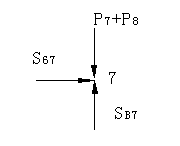Σx=0，   s67=0

Σy=0，   sB7= P7+P8=32.446KN

节点B

Σy=0，  RA- s78- s6Bsin54.46°=0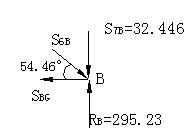Σx=0，   s6Bcos54.46°-sBG=0

∴s6B=322.94KNsBG=187.72KN

节点G

Σx=0，   sGF=sGB=187.72KN

Σy=0，   s6G=0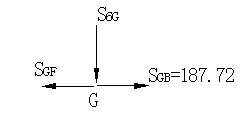Σx=0

s56+ sF6cos54.46°- sB6cos54.46° =0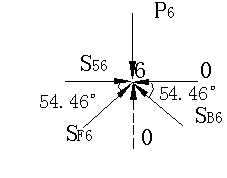Σy=0，  sF6sin54.46°+sB6sin54.46°-P6=0

∴sF6=-211.87KN（负号表示受压）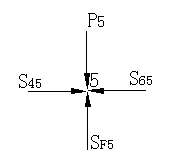s56=310.86KN

节点5

Σx=0，   s45=s65=310.86KN

Σy=0，   s6G= P5=132.47KN

节点F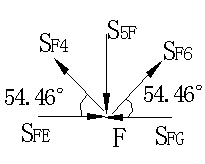Σx=0，

-sFE- sF4cos54.46°+sF6cos54.46°+ sFG =0

Σy=0

sF4sin54.46°- s5F +sF6sin54.46°=0

∴sF4=-49.07KN（负号表示受压）

sFE=339.40KN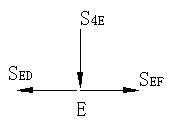节点E

Σx=0，   sED=sEF=339.40KN

Σy=0，   s4E=0KN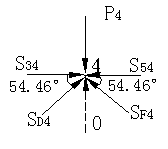节点4

Σx=0，  s34+sD4cos54.46°-s54 +sF4cos54.46°=0

Σy=0，  sD4sin54.46°- P4+sF4sin54.46°=0

∴sD4=101.82KN s34=280.20KN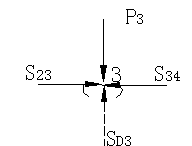节点3

Σx=0，   s23=s34=280.2KN

Σy=0，   sD3= P3=86.28KN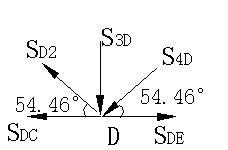Σx=0，

-sDC- sD2cos54.46°-s4Dcos54.46°+ sDF =0

Σy=0

sD2sin54.46°- s3D+s4Dsin54.46°=0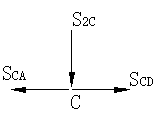∴sD2=207.85KN，  sDC=159.40KN

Σx=0  sCA=sCD=159.4KN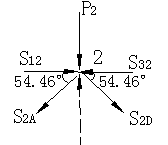Σy=0，   s2C=0KN

Σx=0，

s12- s2Acos54.46°+s2Dcos54.46°- s32=0

Σy=0，   -s2Asin54.46°-s2Dsin54.46°-P2=0

∴s2A=288.75KN，  s12=0KN

σ= sEF/A=339.4×103/2×18.51×104=91.68Mpa[σ]=160Mpa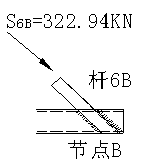A为弦杆截面积，对双[14a槽钢，A=2×18.51cm2

故弦杆强度满足要求。

6B受压力最大，校核焊缝强度

τ=S6B/A= S6B/h×l=322.94×103/0.6×67×10-4=80.33MPa

A—焊缝受剪面积

H—焊脚高度，h=0.6cm

L—焊缝总长，l=14/sin54.46×4=67cm

[τ]=125/1.5=83.33 Mpa

∴τ=80.33[τ] =83.33

3）、横梁校核

、受力分析：平台的计算荷载为P=675KN

经平上的枕木、钢板分配，可假定为均布载荷

q=P/A=675/6.4×4=26.37Kpa

A—平台面积，A=6.4×4m2

、横梁间距0.5m，载荷如下图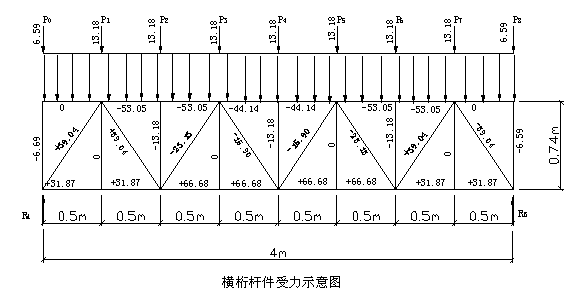、计算节点内力：用节点法计算

、校核

下张杆拉应力G=S/A=66.68×103/9.424×10-4=70.75 Mpa[σ]

A—下张杆截面积，对L70×70×7A=9.424cm2

τ=S/A=39.04×103/2×7×0.6×10-4=46.5 Mpa[τ]=83.3 Mpa

A—剪面积，A=2×70×0.6

4）、结论

（六）、钢梁强度刚度校核

（1、钢梁受力分析：钢梁承受活动平台一侧的轮压力，将平台的重量和工作荷载向钢梁分解。平台自重70KN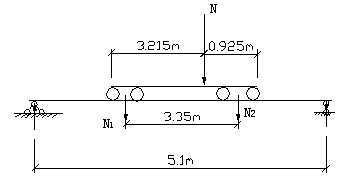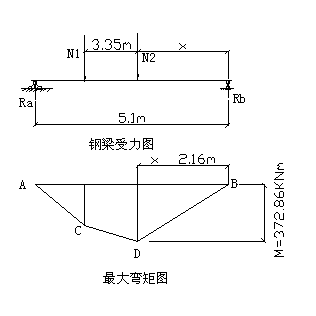C点弯矩方程：Mc＝（63.45+80.24x）×（5.1-3.35-x

＝111.04+76.97x-80.24x2

BD段弯矩方程：Mbd=（345.94-80.24x×x

=345.94x-80.24x2

（2钢梁截面惯性矩和抗弯截面模量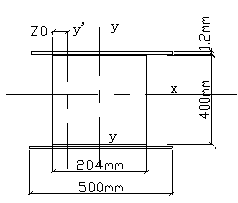（3钢梁强度校核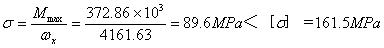（七）、行走轮轮压验算

2PLmax=N2=312.8KN

PLmax =156.4KN

（八）、抓铲机吊装及作业平台移动

2、作业平台移动

㈠、工期计算及劳力配备

1、抓铲机力能计算及工期确定

××下沉深度×虚方系数×回淤超挖系数

31.4×43.6×11×1.2×1.1=19880m3

2、劳力组织

 电工 平台移动操作 班长 机修工 测量 抓铲机司机 潜水员 其他 1人 4人 1人 1 1人 2人 2人 2人

㈡、作业顺序及土方处置

S

 1 2 3 4 5 6 7 8 9 10 11 12 13 14 14 13 12 11 10 9 8 7 6 5 4 3 2 1

N

⑵10→9→8　　      ⑵10→9→8

⑶4→3→2→1　  　  ⑶4→3→2→1

⑷5→6→7　　　     ⑷5→6→7

㈢、 抓铲抓土下沉操作要点

（四）、沉井下沉测量监控及质量控制

（五）、沉井施工常见问题防治办法

1、沉井纠偏

2、沉井下沉过慢或不下沉

3、沉井下沉过快

4、瞬间突沉

(1)在软粘土层中，沉井侧面摩阻力很小，当沉井内抓土较深，或刃脚下土层掏空过多，使沉井失去支撑，常导致突然大量下沉，或急剧下沉。

(2)当粘土层中抓土超过刃脚太深，形成较深锅底，刃脚下的粘土一旦被水浸泡而造成失稳，会引起突然塌陷，使沉井突沉。

遇到此种情况采取的预防措施有：

(1)抓土时，在刃脚部位保留约0.5~1.0m宽的土堤，控制均匀切土，使沉井挤土缓慢下沉。

(2)在粘土层中严格控制抓土深度(一般为40cm)，不能太多，不使挖土超过刃脚，可避免出现深的锅底将刃脚掏空。

5、位移或扭位

1、入冬前对施工机械、设备进行一次全面检查和加固，并做好防寒措施。

2、每日及时了解天气和气温情况，建立生产调度气象记录，做好冬季施工的准备工作，及时采取防降温措施。

3、接到降温、大风及雨雪天气的通知后，及时对抓铲机及活动平台等施工设施进行加固，必要时停止作业。

4、施工机械设备均进行防寒保护，抓铲机因天气原因暂停施工时，将水箱内的水放空并进行标识。

5、做好劳保和防寒用品的发放工作。

6、如果活动平台和抓铲机上有积雪和结冰等情况及时进行清扫。

1、施工中应严格执行国家颁发的《建筑安装工程安全技术规程》和电力建设有关安全的各项规定。

2、起重作业时，必须对工作现场工作环境、行使路线、建筑物以及物件重量等情况进行全面了解。

3、操作人员在进行起重回转、变幅、行走和吊钩升降等动作前，应鸣声示意，严格执行指挥人员信号，特种操作人员必须有特种作业安全操作证。

4、遇有六级以上大风或大雨等恶劣天气时，应停止露天作业。

5、起重机的变幅指示器、力矩限制器以及各种行程限位开关等安全保护装置，必须齐全完整、灵敏可靠，不得随意调整和拆除。

6、起重机卷筒上钢丝绳应连接牢固、排列整齐，放出钢丝绳时卷筒上至少要保留三圈以上，防止钢丝绳打环、纽结、弯折和乱绳，采用绳卡固接时，数量不得少于3个，绳卡滑鞍应在钢丝绳工作时受力一侧，不得正反交错。

7、起重操作人员必须经培训、考试、发证持特种作业人员操作证，方可上岗作业。

8作业前应全部伸出支腿，并采用方木或铁板垫实，调整水平度，锁牢定位销；

9 起重机吊装作业时，汽车驾驶室内不得有人，重物不得超越驾驶室上方且不得在车前区吊装；

10起重机作业时，臂杆吊物回转时动作应缓慢进行；

11 起重机吊物下降时必须采用动力控制，下降停止前应减速，不得采用紧急制动；

12 起重机的安全装置除应按规定装设力矩限制器、超高限位器等安全装置外，还应装设偏斜调整和显示装置。

13、井上施工属高空和水上作业，必须搞好安全防护设施进行安全教育，井上工作人员要搞好个人防护措施，不适宜高空作业和不会游泳者禁止上井。

14、抓斗车，活动平台要可靠固定。

15、按沉井下沉有关施工规范施工，防止井偏，突沉，塌方等造成的安全事故。

1、建立沉井下沉施工现场指挥组，实施现场指挥，确保工程质量、安全和进度。

2、应有专业沉井下沉值班人员指挥施工。

3、在沉井下沉期间，施工人员必须按操作技术要求进行上岗，技术人员要注意观察，根据天气变化情况，控制土质的密实度和摩擦力与土质之间的相应关系，终沉到位井内的土与到位预留量相符，既不能多，但绝不能少，技术人员随时根据历沉曲线决定，要防止沉井突沉和大雨后突沉的现象发生。根据本工程的具体情况，沉井下沉施工中，特别每时每刻应注意的几项要求。下沉的沉井四角载荷不对称，容易发生下沉难度；下沉的沉井四角载荷不等，土的密实度低摩擦力小会影响到向一面倾斜，应引起我们对下沉的注意；终沉始终把关，平稳、对称、均匀、稳固的进行慢沉。

4、沉井下沉时在沉井进入轨道在6m后，必须在相应平稳，纠偏中下沉。

5、沉井终沉时，随时随地注意纠偏、平稳、对称，测量人员必须跟班测量，按下沉曲线放慢下沉速度，确保沉井下沉到位达标。

6、下沉过程中，应做到均匀，对称出土，严格控制泥面高差，当出现平面位置和四角差出现偏差时应及时纠正，纠偏时不可大起大落，避免沉井偏离轴线，同时应注意纠偏幅度不宜过大，频率不宜过高。

7、测量人员必须将测量数及时交当班施工负责人和技术主管，以便及时纠偏或掌握下沉情况。

8、施工时要做好沉井下沉施工记录。

 序号 名     称 规格或型号 单位 数量 备注 1 履带吊 W-1010 台 2 2 抓斗 1.5m3/斗 个 4 备用2个 3 轮胎吊 200t 台 1 4 轮式装载机 ZL50 辆 1 5 反铲挖掘机 WY100 台 1 6 自卸汽车 5t 辆 4 7 电焊机 BX3-400 台 2 8 高压水泵（水枪） 130m3/h 台 2 9 照明灯具 1000W 个 6 10 潜水装备 套 2 外租 11 卷扬机 2t 台 4 12 电子经纬仪 ET-02 台 1 13 水准仪 DS3 台 1